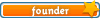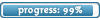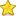Welcome

It is highly recommended that you have Javascript enabled; many features will not work unless you do. The recommended browsers are Firefox and Chrome; the board is also NOT mobile-friendly.

If you were referred by someone (ex. me, Jessica) please put their username in the referral box on the registration page. Ask them if you don't know their username.

If you are visiting for TESTING PURPOSES ONLY, this is the test account information:

Test

Select a forum to post in:

## Ideal Gas Law and Kinetic Theory

Science topics

Moderators: Jessica, Teachers

Looking for a topic?
View the Science Topics Directory to find topics quicker under different kinds of science.

### Ideal Gas Law and Kinetic Theory

Molecular Mass, the Mole, and Avogadro's Number

The relationship between the atomic mass unit and the kilogram isMacroscopic amounts of materials contain large numbers of atoms or molecules. Even in a small volume of gas, 1 cm3, for example, the number is enormous. It is convenient to express such large numbers in terms of a single unit, the gram-mole, or simply the mole (symbol: mol). One gram-mole of a substance contains as many particles (atoms or molecules) as there are atoms in 12 grams of the isotope carbon-12. Experiment shows that 12 grams of carbon-12 contain 6.022 × 1023 atoms. The number of atoms per mole is known as Avogadro's number N[sub]A[/sub], after the Italian scientist Amedeo Avogadro (1776–1856):Thus, the number of moles n contained in any sample is the number of particles N in the sample divided by the number of particles per mole N[sub]A[/sub] (Avogadro's number):The number n of moles contained in a sample can also be found from its mass.Since one gram-mole of a substance contains Avogadro's number of particles (atoms or molecules), the massof a particle (in grams) can be obtained by dividing the mass per mole (in g/mol) by Avogadro's number:• 0Jessica
Board Owner
Topic AuthorSapphirePosts: 3,472
Topics: 1,244
Articles: 30
Joined: December 22nd, 2010, 8:04 pm

### Re: Ideal Gas Law and Kinetic Theory

The Ideal Gas Law

An ideal gas is an idealized model for real gases that have sufficiently low densities. The condition of low density means that the molecules of the gas are so far apart that they do not interact (except during collisions that are effectively elastic). The ideal gas law expresses the relationship between the absolute pressure, the Kelvin temperature, the volume, and the number of moles of the gas.

Ideal Gas Law
The absolute pressure P of an ideal gas is directly proportional to the Kelvin temperature T and the number of moles n of the gas and is inversely proportional to the volume V of the gas:. In other words,where R is the universal gas constant and has the value ofSometimes, it is convenient to express the ideal gas law in terms of the total number of particles N, instead of the number of moles n.The constant termis referred to as Boltzmann's constant, in honor of the Austrian physicist Ludwig Boltzmann (1844–1906), and is represented by the symbol k:With this substitution, the ideal gas law becomes• 0Jessica
Board Owner
Topic AuthorSapphirePosts: 3,472
Topics: 1,244
Articles: 30
Joined: December 22nd, 2010, 8:04 pm